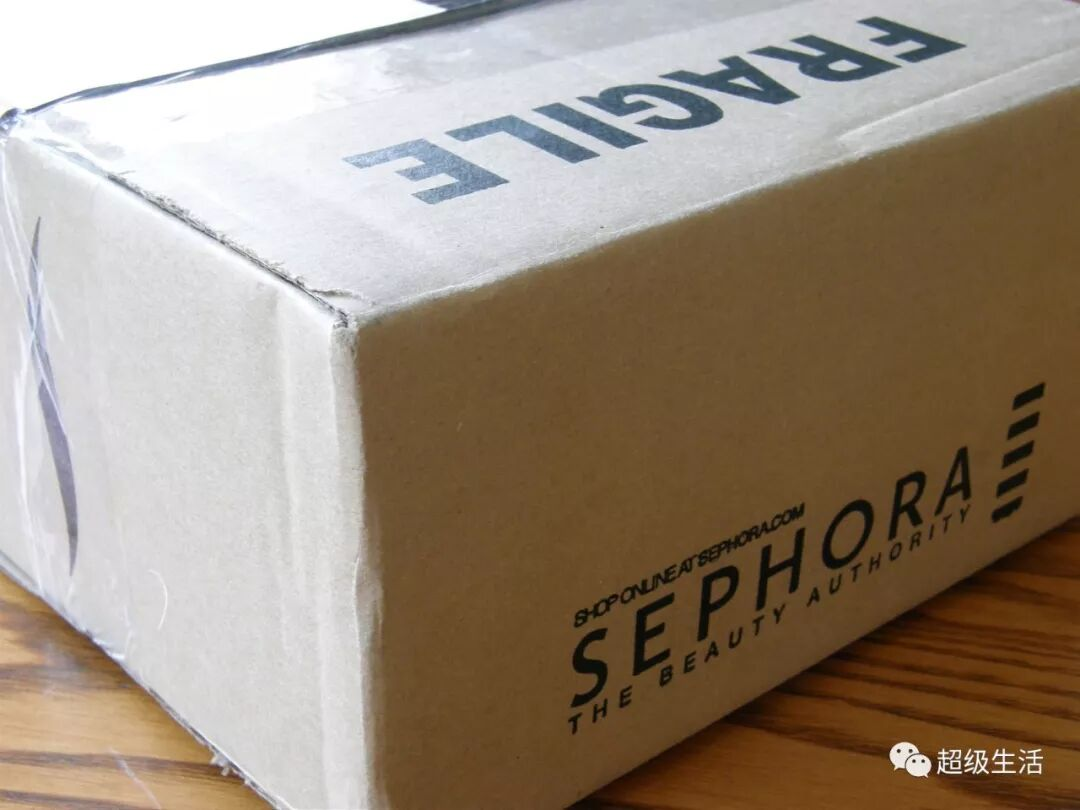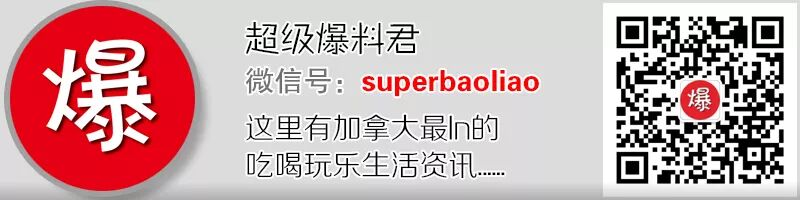2020-01-07 16:31:39

## （超级生活/晓晨）

2020年伊始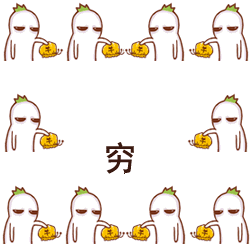JAN

1.>>>健身器材<<<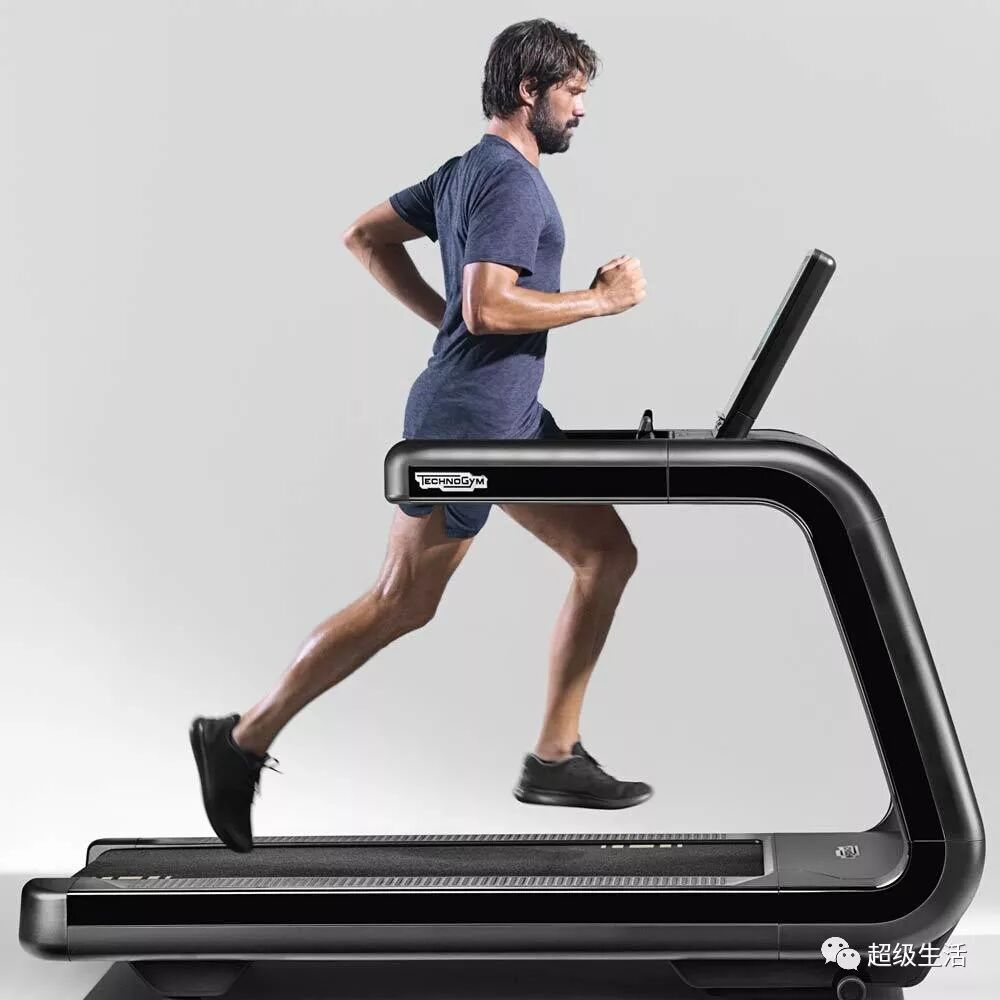2.>>>床上用品<<<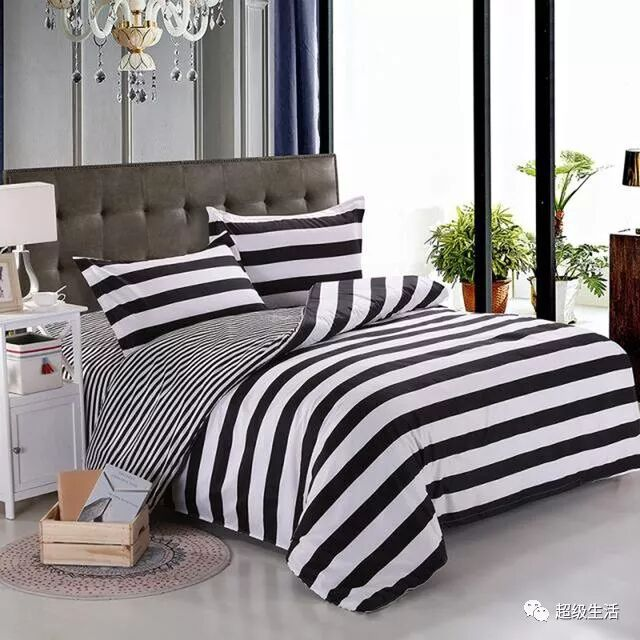3.>>>包装纸，节日饰品<<<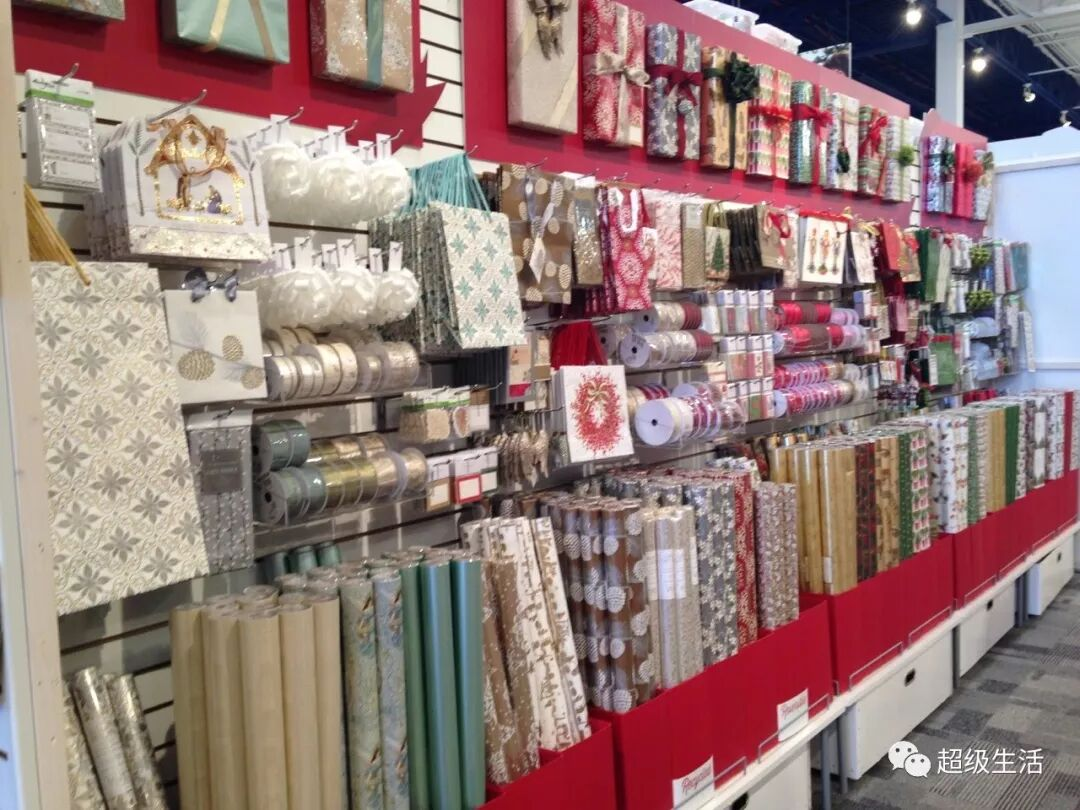FEB

1.>>>冬季外套羽绒服等<<<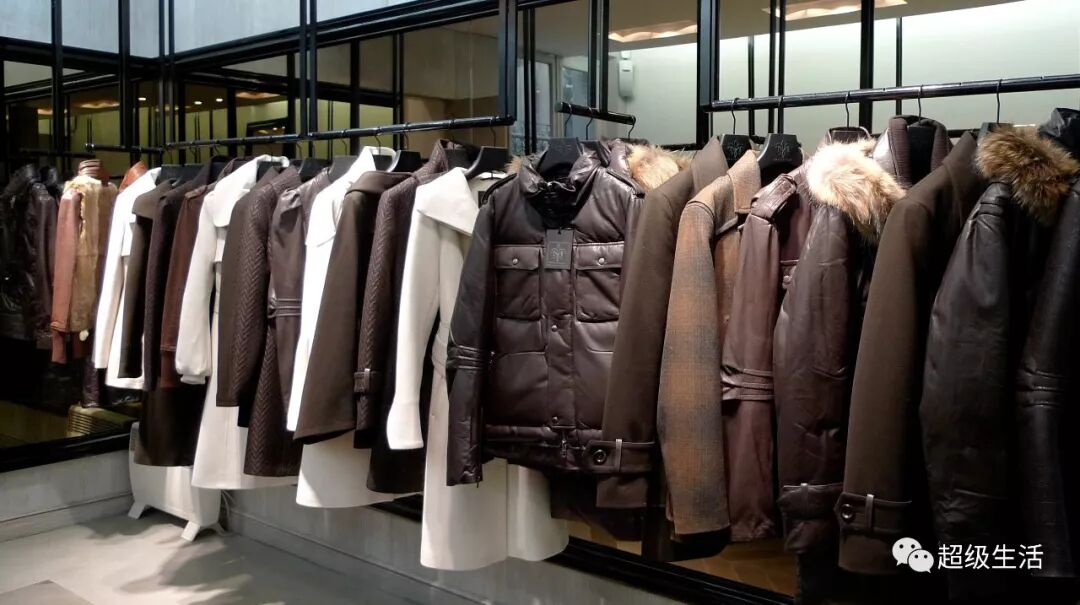2.>>>巧克力<<<3.>>>电视机<<<MAR

1.>>>行李箱<<<2.>>>BBQ烧烤炉<<<3.>>>报税软件<<<APR

1.>>>吸尘器<<<2.>>>旅游度假套餐<<<3.>>>丝芙兰打折<<<MAY

1.>>>家电<<<2.>>>席梦思床垫<<<3.>>>给妈妈的礼物们<<<JUN

1.>>>健身房会员<<<2.>>>内衣<<<3.>>>工具等给爸爸的礼物们<<<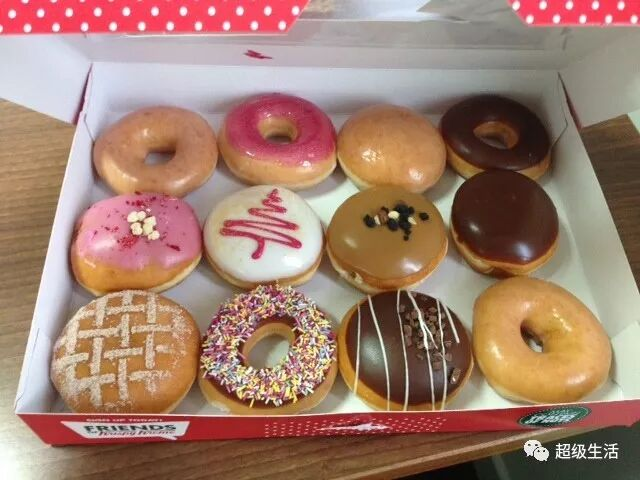JUL

1.>>>服饰<<<7月1日加拿大国庆日的前后都是打折季。各种夏日服饰已经开始清仓了。
2.>>>家具家居用品<<<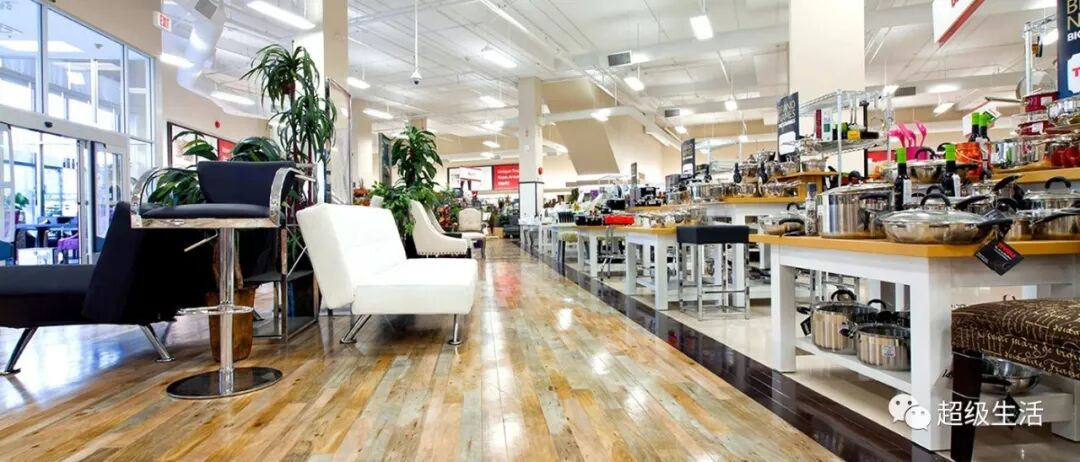3.>>>亚马逊<<<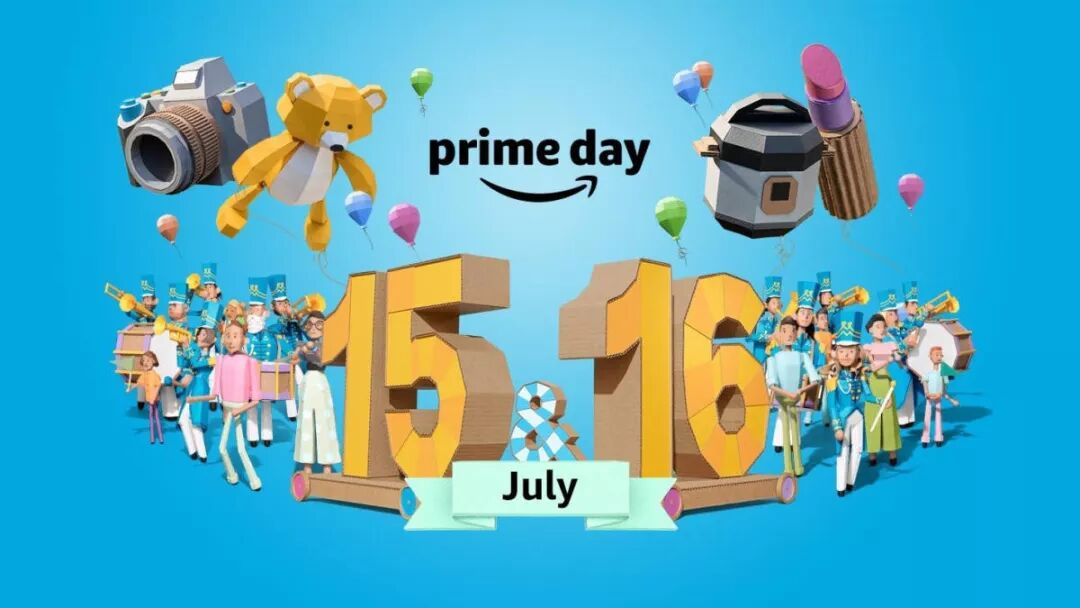AUG

1.>>>泳衣比基尼<<<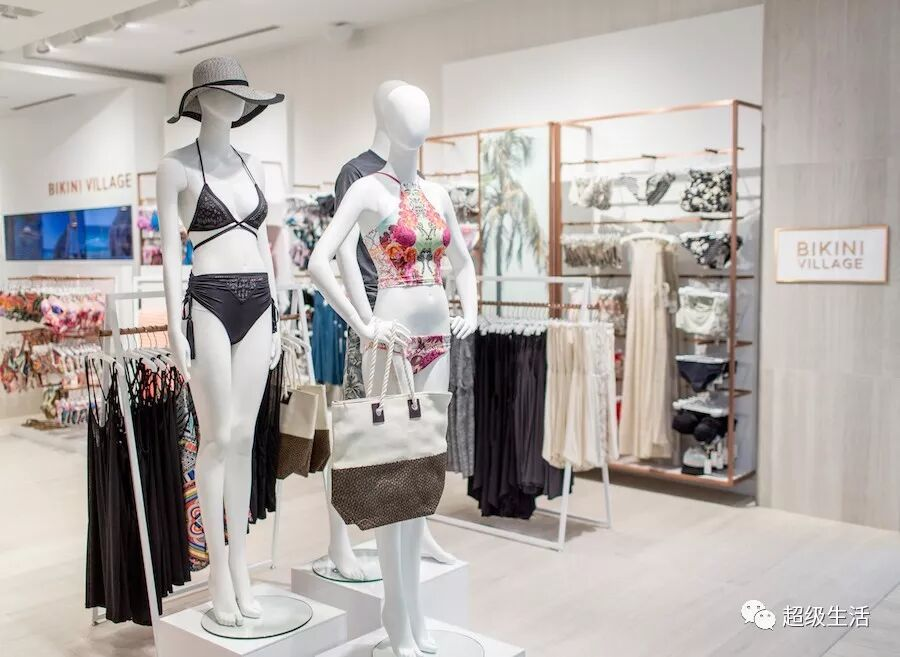2.>>>学生党需要的东西<<<3.>>>苹果电脑<<<Apple近年来每年的返校活动都有买电脑送beats耳机的活动。如果是学生的话那个时候买电脑最划算
SEP

1.>>>各种劳动节促销<<<2.>>>苹果产品<<<3.>>>汽车<<<OCT

1.>>>户外工具/家具<<<2.>>>万圣节糖果<<<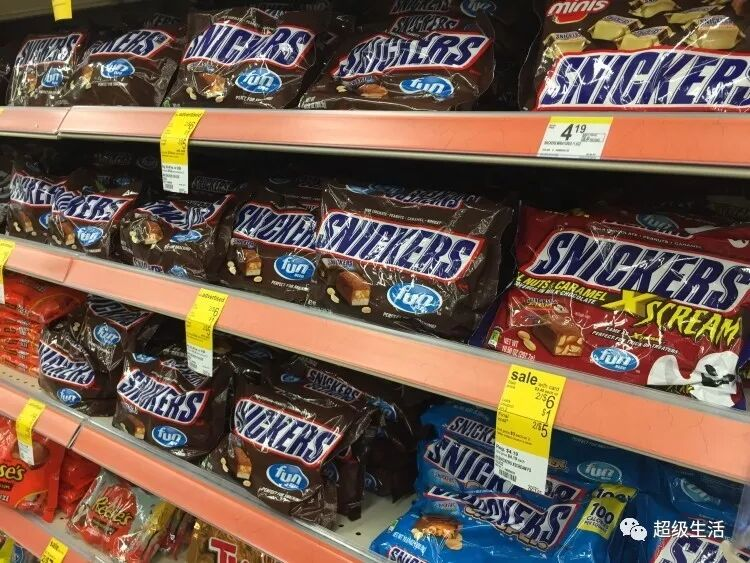3.>>>旅游套餐<<<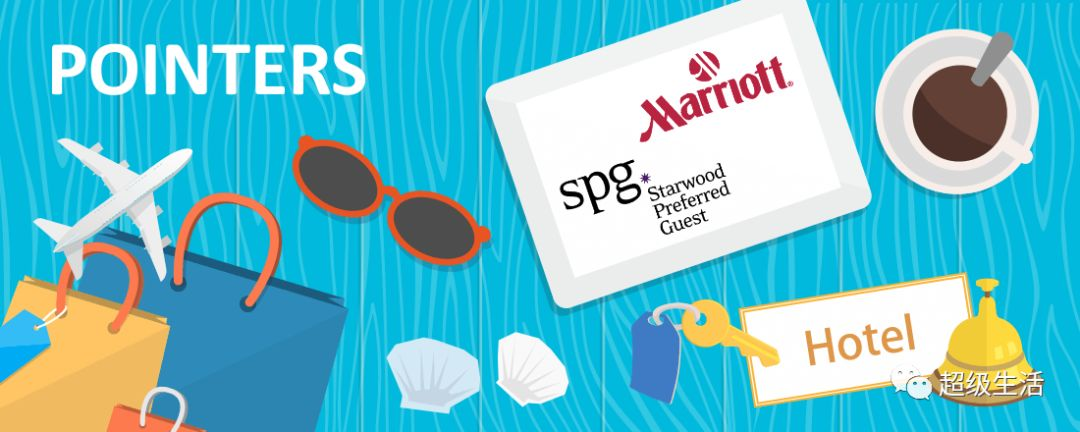1.>>>黑五+网购星期一<<<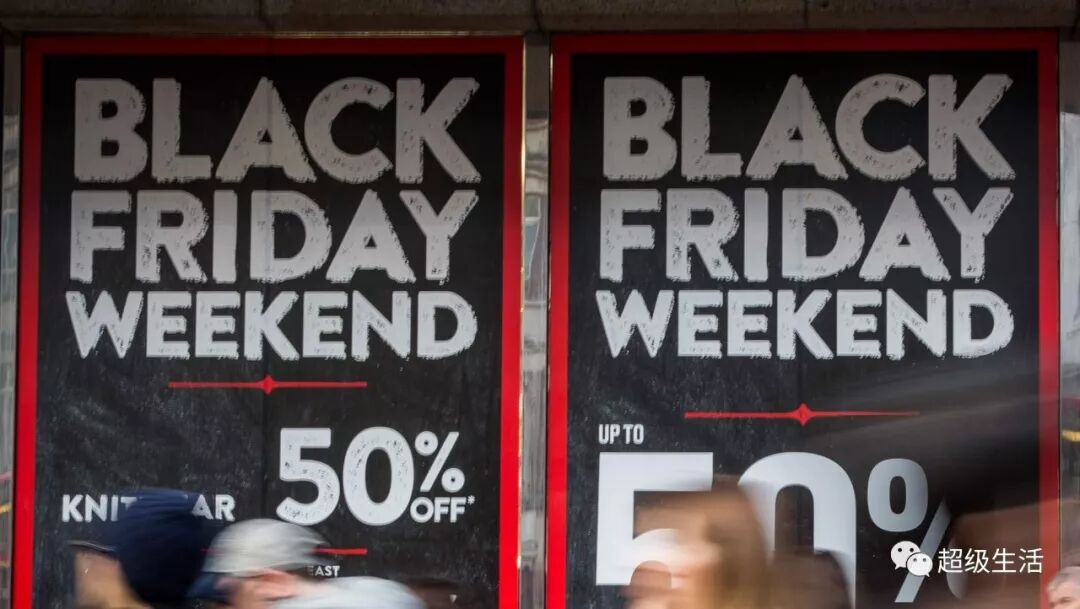2.>>>双十一<<<3.>>>丝芙兰年底打折<<<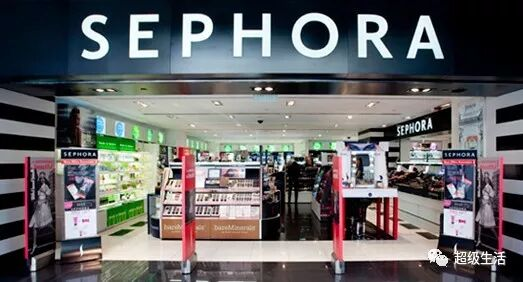DEC

1.>>>圣诞饰品，灯饰<<<2.>>>Boxing Week<<<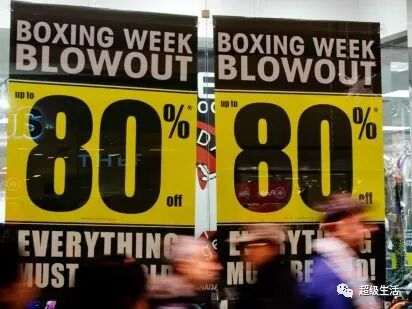>>>丝芙兰最后拼一波<<<